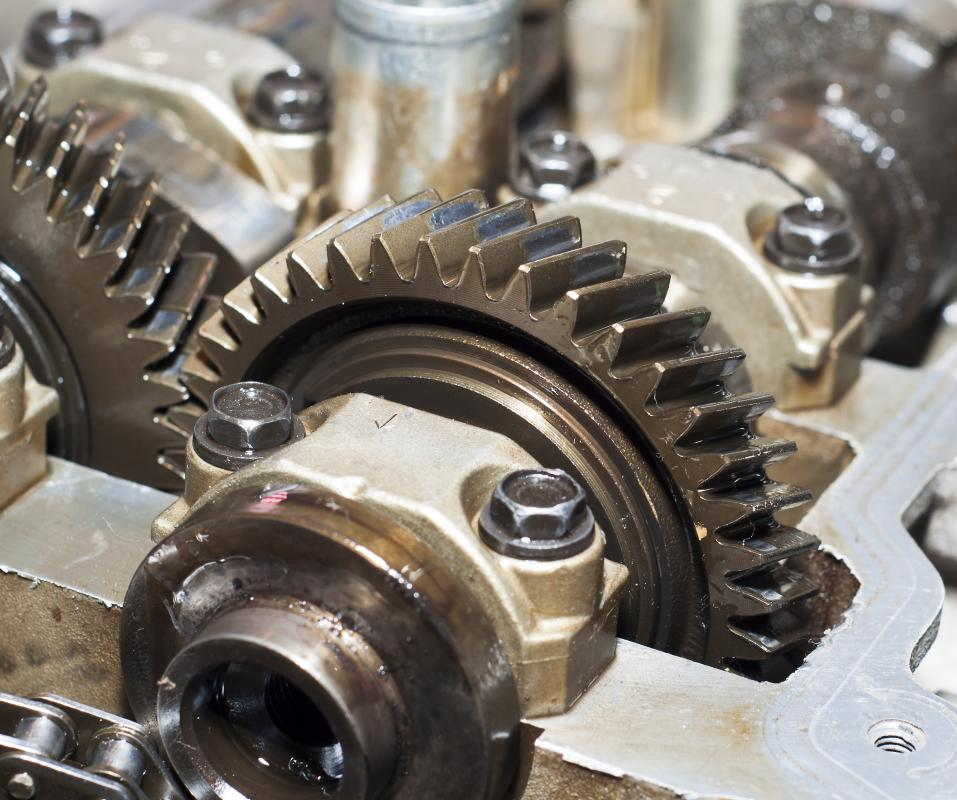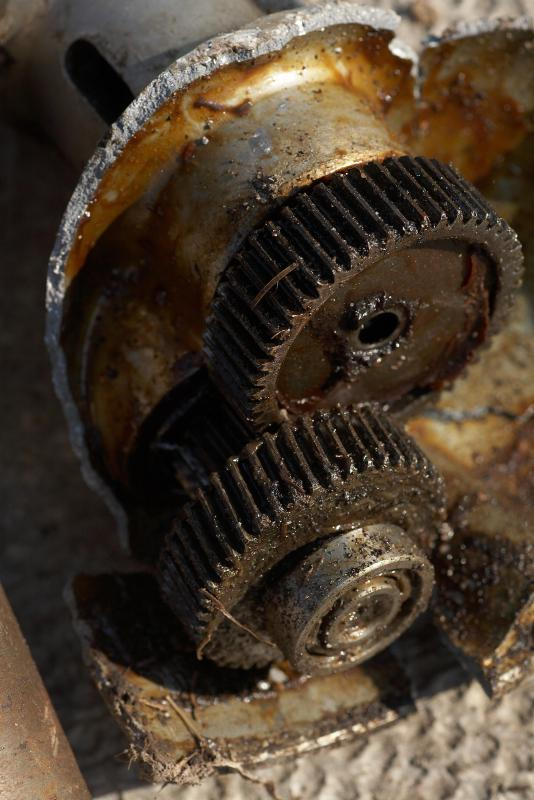# What is a Reduction Gear?

James Back
James BackA reduction gear is a system of gears set up to reduce the speed but increase the strength of an engine or motor.

A reduction gear is part of a mechanical system of gears and shafts that serves the primary purpose of slowing things down within a machine so that energy one place can be transferred and used in another. Reduction gears are most commonly seen in car and truck drivetrains, and in these settings they reduce the high rotational speeds of the engine and convert them to a usable, slower speed that the tires can interpret and safely use. These sorts of gear configurations are also frequently used in heavy machinery and other mechanical appliances both large and small. Anything that uses high rotational speeds in one place but needs energy reduced in another can make use of this sort of internal system. When the gears are working properly, the rotational speed of the input shaft converts to a slower rotational speed on the output shaft. This reduction in output speed helps increase increase torque. The system can look slightly different in different settings, but the concept is usually the same.

#### Basic CompositionAn automobile drivetrain is an example of a multi-stage gear reduction system.

These sorts of gear configurations almost always occur within the inner workings of motors, machines, and other mechanical components. In terms of basics, they aren’t usually very complicated. A simple reduction gear consists of two gears that have the same size teeth but are of different diameters. The number of teeth is proportional to the circumference; the smaller circumference gear will have fewer teeth than the larger one. For example, a gear with a circumference of 16 inches (40.64 cm) will have twice as many teeth as one with a circumference of 8 inches (20.32 cm).Gear ratio is almost always determined by the number of teeth involved.

When these gears mesh in a reduction gearbox, the smaller gear makes two revolutions for every one revolution of the larger gear — in other words, it spins twice as fast. Conversely, the amount of torque available on the larger shaft would be twice as much as that on the smaller. As the speed of the output decreases, the torque is increased proportionally.

#### Determining Gear Ratio

Gear ratio, which is a way of expressing the relationship between the relative velocities of each involved gear, is almost always determined by the number of teeth involved. This in turn is expressed in the ratio of the number of teeth on the larger gear to the number of teeth on the smaller gear. For example, a single-stage gear reduction system consisting of two gears, one with 30 teeth and the other with 10 teeth, would have a gear ratio of 30:10, or 3:1. In this system, the larger gear would turn at one-third the speed of the smaller, while having three times the available torque.

Multi-stage gear reduction gearboxes allow for much higher gear ratios than are practical with single-stage systems. Additional shafts and gears are used in these systems. For example, a small gear is added to the output shaft of the first set of gears to serve as the input driver for a second set of gears. Additional sets of gears can be added as needed. The final gear ratio is determined by multiplying the ratio of each set of gears. For example, a reduction gearbox consisting of three sets of gears with gear ratios of 3:1, 4:1, and 5:1 would yield a final gear ratio of 60:1.

#### Drivetrains as a Common Example

Car drivetrains are a good example of a multi-stage gear reduction system. A typical engine turns at 1500 to 3000 revolutions per minute (RPM), a speed far greater than is needed at the tires. In fact, if this much power were to go to the tires, it would probably cause serious problems since it would likely be more than they could handle. The car’s transmission reduces the RPMs to the driveshaft, sufficiently increasing the torque to move the vehicle. The rear end further reduces the speed to a usable level, while multiplying the amount of torque available to the drive wheels.

Many other machines, including many electrically-powered hand tools, use some form of a reduction gear to control output speed while increasing available torque. This allows small, relatively low-powered electric motors to perform work that would otherwise require much larger, more powerful motors.

## You might also Like